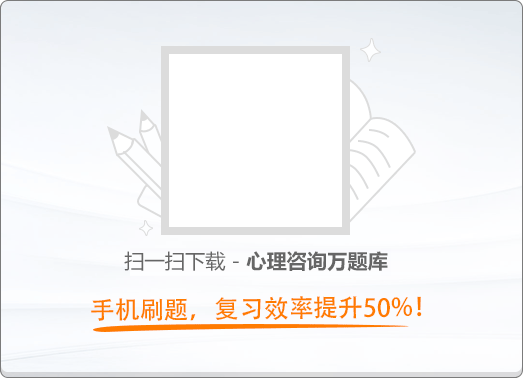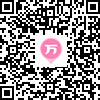90:002015年5月三级心理咨询师《职业道德、理论知识》真题

1
(单项选择题)

• A.

道德是一种社会规范性力量

• B.

道德是领导意志的集中体现

• C.

个体的道德表现差异很大，判定一个人的道德优劣是不可能的

• D.

普遍良好的道德，仅仅是人的善良愿望而已

• A
• B
• C
• D

2
(单项选择题)

• A.

产生的时间晚

• B.

比法律的适用范围广

• C.

内容上显得十分笼统

• D.

评价标准难以确定

• A
• B
• C
• D

3
(单项选择题)

• A.

文明礼貌是企业形象的核心与关键

• B.

企业形象的本质是企业的环境卫生和企业员工的服饰状况

• C.

企业形象是社会公众和企业员工对企业的整体印象和评价

• D.

通过持久、大规模的媒体宣传，就能树立起企业形象

• A
• B
• C
• D

4
(单项选择题)

• A.

企业礼俗

• B.

企业价值观

• C.

企业作风

• D.

规章制度

• A
• B
• C
• D

5
(单项选择题)

• A.

企业存在着无形资产和有形资产两种形式

• B.

人是有形资产，人作为资产通过劳动产生价值

• C.

人是企业发展的决定性因素

• D.

企业的无形资产是一种神秘的物质

• A
• B
• C
• D

6
(单项选择题)

• A.

即使知道领导的决策是错误的，也要不折不扣地执行

• B.

对于领导含糊交办的任务，要含糊执行

• C.

如果不同意领导的意见，要敢于随时说出自己的想法

• D.

一般不越级汇报工作

• A
• B
• C
• D

7
(单项选择题)

• A.

科学发展，高效发展，健康发展

• B.

以科学为本，科学、平稳、顺利发展

• C.

以人为本，全面、协调、可持续发展

• D.

以人为本，科学、高效、健康发展

• A
• B
• C
• D

8
(单项选择题)

• A.

职业劳动是人们无奈的选择

• B.

职业劳动是人们谋生的手段

• C.

职业劳动是市场经济条件下就业竞争加剧的结果

• D.

职业劳动是人生的全部内涵

• A
• B
• C
• D

9
(多项选择题)

• A.

“有完没完”

• B.

“我就这态度”

• C.

“我解决不了，愿意找谁找谁去”

• D.

“后边等着去”

• A
• B
• C
• D

10
(多项选择题)

• A.

职业责任属于道德范畴，而不属于法律范畴

• B.

凡是社会职业，都有明确的职业责任规定

• C.

只有明文规定的职业责任，才必须履行

• D.

职业责任具有一定的强制性

• A
• B
• C
• D

11
(多项选择题)

• A.

个人内在条件

• B.

社会需要

• C.

后天努力程度

• D.

领导赏识

• A
• B
• C
• D

12
(多项选择题)

• A.

真实

• B.

信任

• C.

不欺骗对方

• D.

不欺骗自己

• A
• B
• C
• D

13
(多项选择题)

• A.

某员工利用因特网技术成功下载了竞争对手的设计软件

• B.

某电脑供应商在消费者购买的电脑上安装了盗版的操作软件

• C.

某员工完成某项工作原计划需要8天，实际上用了18天

• D.

某员工在参考别人软件的基础上，制作了本公司的财务软件

• A
• B
• C
• D

14
(多项选择题)

• A.

闲谈莫涉及企业的核心技术

• B.

制作所谓的假秘密散发出去，迷惑竞争对手

• C.

向亲朋好友讲述企业内幕时，要控制在很小的范围内

• D.

企业有危害社会和国家的“秘密”，要敢于揭露

• A
• B
• C
• D

15
(多项选择题)

• A.

真理往往掌握在少数人手中，要树立相信少数人的观念

• B.

书本知识往往是错误的，破除本本主义，不再相信书本知识

• C.

老师的话往往不一定正确，要敢于对老师得出的结论提出质疑

• D.

树立实践观点，坚持实践是检验真理的唯一标准

• A
• B
• C
• D

16
(多项选择题)

• A.

节俭纯属个人之事，不适宜作为普遍性的要求

• B.

节俭是物质短缺时代的特殊要求，在物质产品充裕情况下无须节俭

• C.

节俭是安邦定国的法宝，因为国家的发展进步时时需要节俭

• D.

节俭作为一种美德，不应以财富多寡作为评价的前提

• A
• B
• C
• D